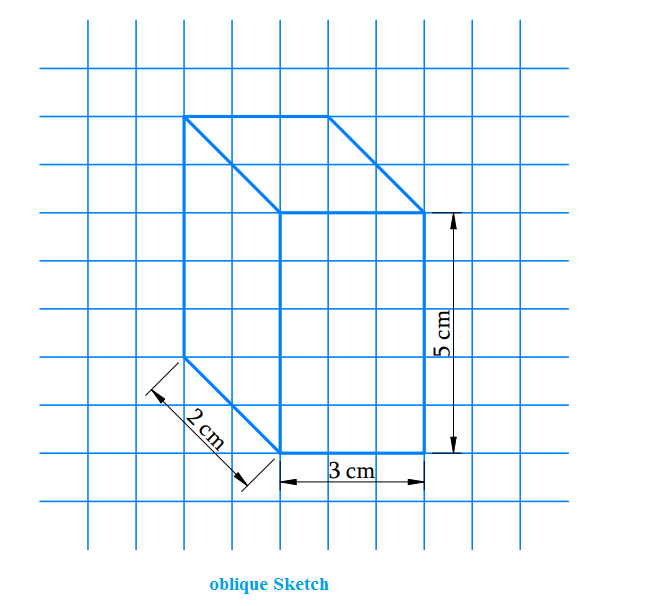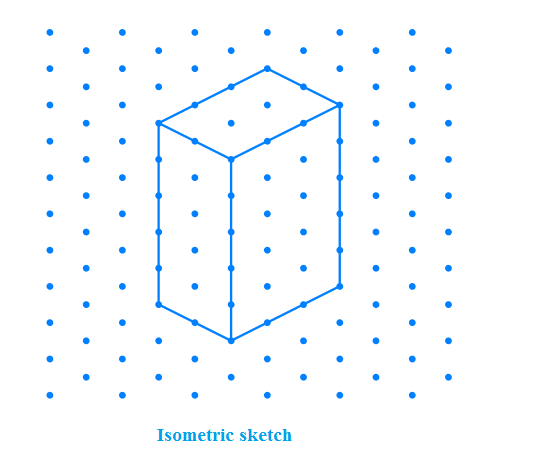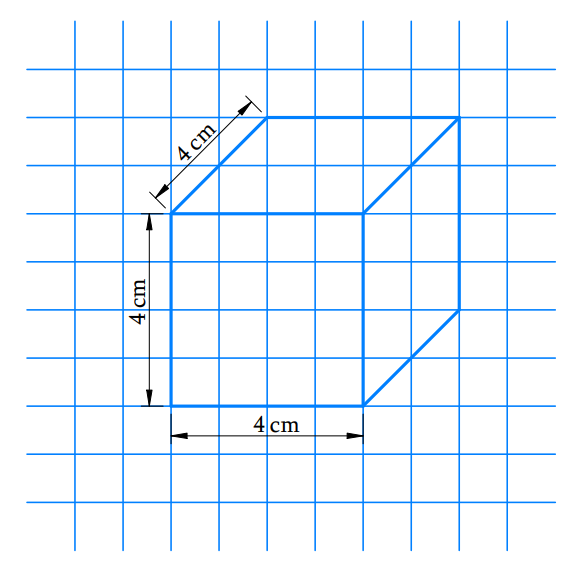# Ex.15.2 Q5 Visualising Solid Shapes - NCERT Maths Class 7

## Question

Give (i) an oblique sketch and (ii) an isometric sketch for each of the following:

(a) A cuboid of dimensions $$5$$ cm, $$3$$ cm and $$2$$ cm. (Is your sketch unique?)

(b) A cube with an edge $$4$$ cm long.

An isometric sheet is attached at the end of the book. You could try to make on it some cubes or cuboids of dimensions specified by your friend.

Video Solution
Visualizing Solid Shapes
Ex 15.2 | Question 5

## Text Solution

What is known?

Dimensions of the cube and cuboid.

What is unknown?

Oblique and isometric sketch of cube and cuboid.

Reasoning:

First draw a rough sketch then draw oblique and isometric sketch for cube and cuboid.

Steps:

a) oblique and isometric sketch for a cuboid of dimensions $$5$$ cm, $$3$$ cm and $$2$$ cmb) oblique and isometric sketch for a cube with  $$4$$ cm lengthLearn from the best math teachers and top your exams

• Live one on one classroom and doubt clearing
• Practice worksheets in and after class for conceptual clarity
• Personalized curriculum to keep up with school